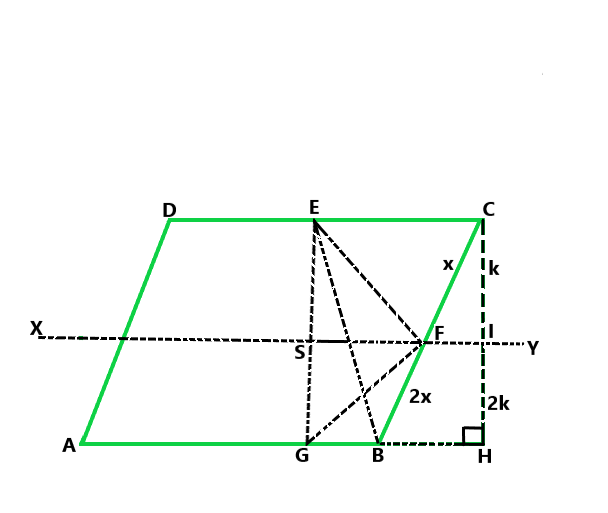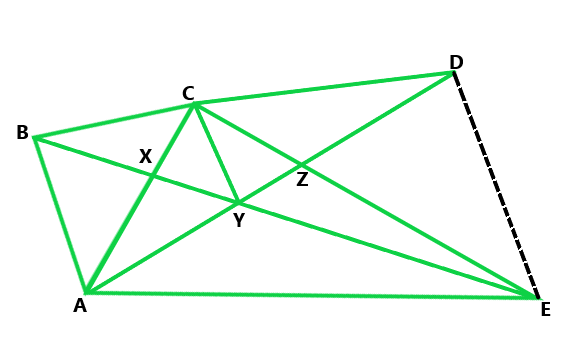GeeksforGeeks App
Open AppBrowser
Continue

## Related Articles

• RD Sharma Class 9 Solutions

# Class 9 RD Sharma Solutions – Chapter 15 Areas of Parallelograms and Triangles- Exercise 15.3 | Set 2

### Question11. If P is any point in the interior of a parallelogram ABCD, then prove that area of the triangle APB is less than half the area of parallelogram.

Solution:

According to the question

ABCD is a parallelogram and P is any point in the interior of parallelogram

Prove: ar(ΔAPB) < 1/2 ar(|| gm ABCD)

Proof:

Construction: Draw DN ⊥ AB and PM ⊥ AB

Now, we find the area of ∥gm ABCD

= AB × DN,

Now, the area of ΔAPB

= (1/2) (AB × PM)

Now, PM < DN

AB × PM < AB × DN

(1/2)(AB × PM) < (1/2)(AB × DN)

ar(ΔAPB) < 1/2 ar(∥ gm ABCD)

Hence proved

### Question 12. If AD is a median of a triangle ABC, then prove that triangles ADB and ADC are equal in area. If G is the mid-point of the median AD, prove that ar(ΔBGC) = 2ar(ΔAGC).

Solution:

According to the question

AD is a median of a triangle ABC

Now, construct AM ⊥ BC

It is given that AD is the median of ΔABC

So, BD = DC

BD = AM = DC × AM

(1/2)(BD × AM) = (1/2)(DC × AM)

ar(ΔABD) = ar(ΔACD)                 ….(i)

In ΔBGC,

GD is the median

So, ar(ΔBGD) = ar(ΔCGD)          …..(ii)

In ΔACD,

CG is the median

So, ar(ΔAGC) = ar(ΔCGD)        ……..(iii)

From equation (ii) and (iii), we get

ar(ΔBGD) = ar(ΔAGC)

But, ar(ΔBGC) = 2ar(ΔBGD)

Hence, ar(ΔBGC) = 2ar(ΔAGC)

Hence proved

### Question 13. A point D is taken on the side BC of a ΔABC, such that BD = 2DC. Prove that ar(ΔABD) = 2ar(ΔADC).

Solution:

According to the question

BD = 2DC(In ΔABC)

Proof:

Now draw a point E on BD such that BE = ED

Since, BE = ED and BD = 2 DC

So, BE = ED = DC

As we know that, in triangles, median divides the triangle into two equal triangles.

In ΔABD,

AE is the median.

So, ar(ΔABD) = 2ar(ΔAED)            …..(i)

In ΔAEC,

From eq(i) and (ii), we get

Hence proved

### (ii) ar(ΔABP) = 2ar(ΔCBP).

Solution:

According to the question

ABCD is the parallelogram, whose diagonals intersect at O

So, AO = OC and BO = OD

(i) Prove that ar(ΔADO) = ar(ΔCDO)

Proof:

In ΔDAC,

DO is a median.

Hence proved

(ii) Prove that ar(ΔABP) = 2ar(ΔCBP)

Proof:

In ΔBAC,

BO is a median.

So, ar(ΔBAO) = ar(ΔBCO)       …..(i)

In ΔPAC,

PO is a median.

So, ar(ΔPAO) = ar(ΔPCO)          …..(ii)

Now, subtract eq(ii) from (i), we get

ar(ΔBAO) − ar(ΔPAO) = ar(ΔBCO) − ar(ΔPCO)

ar(ΔABP) = 2ar(ΔCBP)

Hence proved

### (ii) If the area of ΔDFB = 3 cm2, find the area of ∥ gm ABCD.

Solution:

According to the question

ABCD is a parallelogram in which BC is produced to E

such that CE = BC and AE intersects CD at F

(i) Prove that ar(ΔADF) = ar(ΔECF)

Proof:

∠ADF = ∠ECF                     (Alternate interior angles)

∠DFA = ∠CFA                      (Vertically opposite angle)

So, by AAS congruence

By c.p.c.t

DF = CF

Hence proved

(ii) From the question area of ΔDFB = 3 cm2

Find the area of ||gm ABCD

Now, DF = CF (Proved above)

BF is a median in Δ BCD.

ar(ΔBCD) = 2ar(ΔDFB)

ar(ΔBCD) = 2 × 3 cm2 = 6 cm2

Now we find the area of a parallelogram = 2ar(ΔBCD)

= 2 × 6 cm2 = 12 cm2

Hence the area of parallelogram is 12 cm2

### Question 16. ABCD is a parallelogram whose diagonals AC and BD intersect at O. A line through O intersects AB at P and DC at Q. Prove that ar(ΔPOA) = ar(ΔQOC).

Solution:

Prove: ar(ΔPOA) = ar(ΔQOC)

In ΔPOA and QOC,

∠AOP = ∠COQ  (Vertically opposite angle)

AO = OC

∠PAC = ∠QCA   (Alternate angle)

So, by ASA congruence criterion, we have

ΔPOA ≅ ΔQOC

Hence ar(ΔPOA) = ar(ΔQOC)

Hence proved

### Question 17. ABCD is a parallelogram. E is a point on BA such that BE = 2EA and F is point on DC such that DF = 2FC. Prove that AECF is a parallelogram whose area is one third of the area of parallelogram ABCD.

Solution:

Prove: ar(∥gm AECF) = 1/3 ar(||gm ABCD)

Proof:

First construct FG ⊥ AB

Now, according to the question

BE = 2 EA and DF = 2FC

AB – AE = 2 AE and DC – FC = 2 FC

AB = 3 AE and DC = 3 FC

AE = (1/3) AB and FC = (1/3)DC            …….(i)

But AB = DC

So, AE = FC                       (Opposite sides of the parallelogram)

AE = FC and AE ∥ FC

So, AECF is a parallelogram

Now, we find the area of parallelogram AECF

= AE × FG

Put the value of AE from eq(i), we get

ar(||gm AECF) = 1/3 AB × FG

3ar (||gm AECF) = AB × FG          ….(ii)

and ar(||gm ABCD) = AB × FG            ….(iii)

From eq(ii) and (iii), we get

3ar(||gm AECF) = ar(||gm ABCD)

Hence ar(||gm AECF) = 1/3 ar(||gm ABCD)

Hence proved

### (iii) ar(ΔRQC) = 3/8 ar(ΔABC).

Solution:

According to the question

ABC is a triangle, in which P, Q, and R are the mid-points of AB, BC and AP

(i) Prove that ar(ΔPBQ) = ar(ΔARC)

Proof:

CR is the median of ΔCAP

So, ar(ΔCRA) = (1/2) ar(ΔCAP)                ….(i)

CP is the median of a ΔCAB

So, ar(ΔCAP) = ar(ΔCPB)                 ….(ii)

So, from eq(i) and (ii), we conclude that

ar(ΔARC) = (1/2) ar(ΔCPB)                     ….(iii)

Now, PQ is the median of a ΔPBC

So, ar(ΔCPB) = 2ar(ΔPBQ)         ….(iv)

From eq(iii) and (iv),  we conclude that

ar(ΔARC) = ar(ΔPBQ)                ….(v)

Hence proved

(ii) Now QP and QR are the medians of triangles QAB and QAP

So, ar(ΔQAP) = ar(ΔQBP)                    ….(vi)

And ar(ΔQAP) = 2ar(ΔQRP)                ….(vii)

From eq(vi) and (vii),  we conclude that

ar(ΔPRQ) = (1/2) ar(ΔPBQ)                  ….(viii)

And from eq (v) and (viii), we conclude that

ar(ΔPRQ) = (1/2) ar(ΔARC)

Hence proved

(iii) Now, LR is a median of ΔCAP

= 1/2 × (1/2) ar(ΔABC)

= (1/4) ar(ΔABC)

and RQ is the median of ΔRBC.

So, ar(ΔRQC) = (1/2) ar(ΔRBC)

= (1/2) {ar(ΔABC) − ar(ΔARC)}

= (1/2) {ar(ΔABC) – (1/4) ar(ΔABC)}

= (3/8) ar(ΔABC)

Hence proved

### Question 19. ABCD is a parallelogram. G is a point on AB such that AG = 2GB and E is point on DC such that CE = 2DE and F is the point of BC such that BF = 2FC. Prove that:

(ii) ar(ΔEGB) = (1/6) ar(ABCD)

(iii) ar(ΔEFC) = (1/2) ar(ΔEBF)

(iv) ar(ΔEGB) = 3/2 × ar(ΔEFC)

(v) Find what portion of the area of parallelogram is the area of ΔEFG.

Solution:

According to the question

ABCD is a parallelogram

So, AG = 2 GB, CE = 2 DE and BF = 2 FC

Now, draw a parallel line to AB that pass through point F and a perpendicular line to AB from C.(i) Prove that ar(ADEG) = ar(GBCE)

Since it is given that ABCD is a parallelogram

So, AB = CD and AD = BC

Now, let us consider the two trapezium ADEG and GBCE

Since AB = DC, EC = 2DE, AG = 2GB

So, ED = (1/3) CD = (1/3) AB and EC = (2/3) CD = (2/3) AB

AG = (2/3) AB and BG = (1/3) AB

So, DE + AG = (1/3) AB + (2/3) AB = AB and EC + BG = (2/3) AB + (1/3) AB = AB

Since these two trapeziums have same heights, also their sum of two parallel sides are equal,

So, the area of trapezium = sum of parallel side/2 x height

Hence proved

(ii) Prove that ar(ΔEGB) = (1/6) ar(ABCD)

From the above we know that

BG = (1/2) AB

So, ar(ΔEGB) = (1/2) × GB × height

ar(ΔEGB) = (1/2) × (1/3) × AB × height

ar(ΔEGB) = (1/6) × AB × height

Then, ar(ΔEGB) = (1/6) ar(ABCD)

Hence proved

(iii) Prove that ar(ΔEFC) = (1/2) ar(ΔEBF)

As we know that the height of triangle EFC and EBF are equal,

So, ar(ΔEFC) = (1/2) × FC × height

ar(ΔEFC) = (1/2) × (1/2) × FB × height

ar(ΔEFC) = (1/2) ar(EBF)

Then, ar(ΔEFC) = (1/2) ar(ΔEBF)

Hence proved

(iv) Prove that ar(ΔEGB) = 3/2 × ar(ΔEFC)

Let us consider the trapezium EGBC

So, ar(EGBC) = ar(ΔEGB) + ar(ΔEBF) + ar(ΔEFC)

Now put all these values, we get

(1/2) ar(ABCD) = (1/6) ar(ABCD) + 2ar(ΔEFC) + ar(ΔEFC)

(1/3) ar(ABCD) = 3 ar(ΔEFC)

ar(ΔEFC) = (1/9) ar(ABCD)

Now from option (ii), we get,

ar(ΔEGB) = (1/6) ar(ΔEFC)

ar(ΔEGB) = (3/2) × (1/9) ar(ABCD)

ar(ΔEGB) = (3/2) ar(ΔEFC)

Hence, ar(ΔEGB) = (3/2) ar(ΔEFC)

Hence proved

(v) From the figure, we have FB = 2CF,

So, Let us considered CF = x and FB = 2x.

Now, we take triangle CFI and CBH which are similar triangle.

So, by the property of similar triangle, we get

CI = k and IH = 2k

Now, we take ΔEGF in which,

ar(ΔEFG) = ar(ΔESF) + ar(ΔSGF)

ar(ΔEFG) = (1/2) SF × k + (1/2) SF × 2k

ar(ΔEFG) = (3/2) SF × k             …….(i)

Now,

ar(ΔEGBC) = ar(SGBF) + ar(ESFC)

ar(ΔEGBC) = (1/2)(SF + GB) × 2k + (1/2)(SF + EC) × k

ar(ΔEGBC) = (3/2) k × SF + (GB + (1/2)EC) × k

ar(ΔEGBC) = (3/2) k × SF + (1/3 AB + (1/2) × (2/3) AB) × k

(1/2) ar(ΔABCD) = (3/2) k × SF + (2/3) AB × k

ar(ΔABCD) = 3k × SF + (4/3) AB × k

ar(ΔABCD) = 3k × SF + 4/9 ar(ABCD)

k × SF = (5/27)ar(ABCD)                …….(ii)

From eq(i) and (ii), we conclude that

ar(ΔEFG) = (3/2) × (5/27) ar(ABCD)

ar(ΔEFG) = (5/18) ar(ABCD)

Hence proved

### (iii) Prove that ar(BCZY) = ar(ΔEDZ)Solution :

According to the question

CD || AE and CY || BA

(i) Here, triangle BCA and triangle BYA are on the same base BA and

between same parallel lines BA and CY.

So, ar(ΔBCA) = ar(ΔBYA)       ….(i)

Now as we know that ar(ΔBCA) = ar(ΔCBX) + ar(ΔBXA)

ar(ΔBYA) = ar(ΔBXA) + ar(ΔAXY)

So, put all these values in eq(i), we get

ar(ΔCBX) + ar(ΔBXA) = ar(ΔBXA) + ar(ΔAXY)

So, ar(ΔCBX) = ar(ΔAXY)

(ii) Here, triangles ACE and ADE are on the same base AE and

between same parallels CD and AE

Now as we know that ar(ΔACE) = ar(ΔCZA) + ar(ΔAZE)

and ar(ΔADE) = ar(ΔAZE) + ar(ΔDZE)

So, put all these values in eq(ii), we get

ar(ΔCZA) + ar(ΔAZE) = ar(ΔAZE) + ar(ΔDZE)

ar(ΔCZA) = ar(ΔDZE)                  ……(iii)

Hence proved

(iii) As we know that ar(ΔCBX) = ar(ΔAXY)

Now, add ar(ΔCYG) on both sides, we get

ar(ΔCBX) + ar(ΔCYZ) = ar(ΔCAY) + ar(ΔCYZ)

ar(BCZY) = ar(ΔCZA)                ….(iv)

From eq(iii) and (iv), we conclude that

ar(BCZY) = ar(ΔDZE)

Hence proved

My Personal Notes arrow_drop_up
Related Tutorials